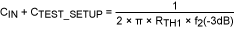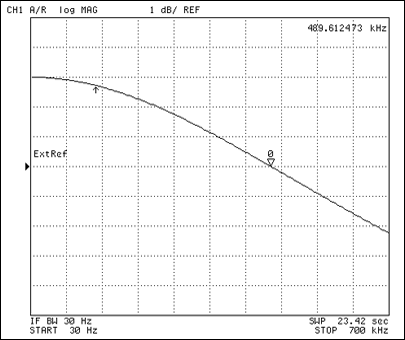# Measure the Input Capacitance of an Op Amp

### Abstract

Op amps with low input capacitance are required in applications such as smoke detectors, photodiode transimpedance amplifiers, medical instrumentation, industrial control systems, and the piezo-sensor interface. Minimizing input capacitance can also increase the frequency of a pole in the feedback path until it has a negligible effect on a circuit. Measuring the input capacitance of an op amp is not trivial, especially if the value is only a few picofarads. Such low values also present difficulties in screening the op amps during production testing. This design idea explains how to accurately determine the input capacitance for any op amp.

A similar version of this article appeared in the May 8, 2011 issue of EE Times magazine.

Op amps with low input capacitance are required in applications such as smoke detectors, photodiode transimpedance amplifiers, medical instrumentation, industrial control systems, and the piezo-sensor interface. CMOS-input op amps, for instance, require minimal input capacitance when amplifying capacitive-sensor outputs or the small signals from high-impedance sources. Input capacitance also affects a pole in the feedback path that can cause instability in high-gain, high-frequency applications. By minimizing this input capacitance, you may be able to increase the corresponding pole frequency until it has a negligible effect on the circuit.

Measuring the input capacitance of an op amp is not trivial, especially if the value is only a few picofarads. Such low values also present difficulties in screening the op amps during production testing. Hence, semiconductor companies often provide only typical values for this parameter, using simulation results and bench measurements on a few known good units. The following discussion can provide a sanity check in the lab by assisting the system-level designer or QA engineer to accurately determine the input capacitance for any op amp.

The direct approach of observing input capacitance on a multimeter is not practical below a few nanofarads. A simple yet effective alternative is to insert a large resistor in series with the op-amp input (Figure 1). Plotting the frequency response of the resulting first-order lowpass RC filter on a network analyzer (i.e., a Bode plot) lets you calculate the op amp's input capacitance. Sounds simple, but you must follow precautions to ensure that the measurement accuracy is not compromised by stray capacitance in the PC board and the test setup.Follow these tips to minimize stray parasitics: Increase the measurement resolution by using only low-capacitance FET probes (< 1pF), such as the Tektronix® P6245. If the series resistor is a surface-mount component, ensure that the board capacitance to ground is as low as possible. This implies no ground-plane layer beneath the input signal traces and the series resistor. If the series resistor is a through-hole component, bend the input pin so it does not contact the PCB, and use a short lead length to solder the resistor directly to the op-amp input pin. Do not use a breadboard in the test setup, because capacitance between the breadboard tracks and jumper wires can degrade the measurement accuracy. Use short traces at the input to minimize series inductance.

The hardware recommended for this test setup (Figure 2) includes an Agilent® 4395A network analyzer, a Mini-Circuits® ZFRSC-2050 power splitter, and a Tektronix P6245 active FET probe.First, calibrate the setup with no op amp installed on the PCB. From the resulting Bode plot, you can calculate stray capacitance aswhere f1(-3dB) is the corner frequency as measured on the network analyzer with no op amp installed, and RTH1 is the Thévenin-equivalent series resistance. RTH1 is a function of the inserted series resistor, the input termination resistance (50Ω), and the source impedance at the power splitter (50Ω):

RTH1 = RSERIES + (50||50)

Next, install the op amp on the PCB. Since the board's stray capacitance is in parallel with the op amp's input capacitance, Equation 1 becomeswhere f2(-3dB) is the corner frequency as measured on the spectrum analyzer with the op amp installed, and RTH2 is the Thévenin-equivalent series resistance. This Thévenin-equivalent resistance is a function of the inserted series resistor, the input termination resistance (50Ω), output impedance of the power splitter (50Ω), and the common mode input impedance of the op amp:

RTH2 = (RSERIES + (50||50))||RCM

The input common-mode impedance of an op amp is not accurately known. For a CMOS-input op amp, however, it is fairly easy to select RSERIES << RCM. Then RTH2 ≈ RTH1, and Equation 3 can be rewritten asYou can now calculate the op amp's input capacitance from Equations 1 and 4, and verify the value by repeating the experiment with two different values of series resistor.

To illustrate the method, consider an input-capacitance measurement for the MAX4238 op amp. Figure 3 shows the amplitude response from Figure 2 using a 200kΩ series resistor and no op amp installed on the PCB, and Figure 4 shows the amplitude response with the MAX4238 installed.Table 1 summarizes the results, using the frequency-response waveforms and calculations from Equations 1 and 4. As a sanity check, the measurement was repeated with a different series resistor value to demonstrate that a similar result (≈ 4pF) is obtained.

 RSERIES (kΩ) f1(-3dB) (kHz) f2(-3dB) (kHz) CTEST SETUP (pF) CIN (pF) 199.4 489.67 144.96 1.63 3.86 64.2 1475 471.5 1.68 3.62